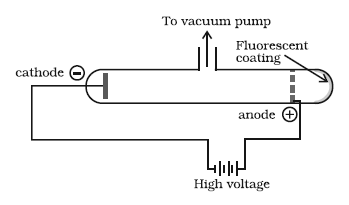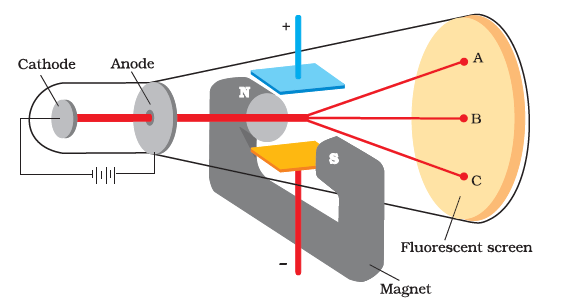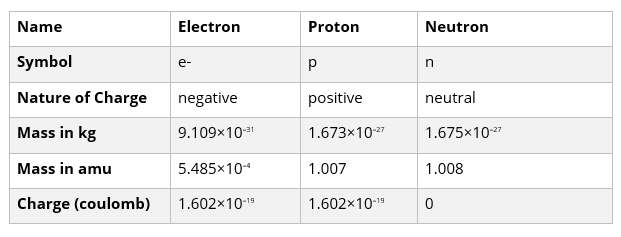# STRUCTURE OF ATOM

## Structure Of Atom

Atoms consists of Electron, proton and Neutrons. We will see how these particles were discovered

## Discovery Of Electron – Discharged tube experiment

• William Crook studied the conduction of electricity through a gas at low pressure.
• In the experiment the electrical discharge tube is filled with gas at low pressure & high voltage.
• When high voltage is applied across the electronic current start flowing through stream of parts moving in the tube from cathode (-) to anode (+). These rays are known as cathode and anode rays.
Properties of Cathode rays:-
1. They travel in a straight line.
2. They start from cathode and move towards anode.
3. These rays are not visible but their behavior can be observed with fluorescent material which glow when hit by them
4. In absence of electrical or magnetic field cathode rays travel in straight lines
5. In presence of electrical or magnetic field, behavior of cathode rays is like that shown by negatively charged particles suggesting that cathode rays consist of negatively charged particles called electrons
6. The characteristics of cathode ray do not depend upon material of electrode and not of gas.It was concluded from the experiment that electron are basic constituent of matter
Determination of Ratio: - (Charge mass ratio)1. J. J. Thomson firstly determined Ratio for electrons.
2. The subjected beam of electrons/ cathode ray to influence electric and magnetic field. These were adding perpendicular to each other as well as the path followed by electron.
3. J. J. Thomson carry out accurate measurements for the deflections shown by electrons. he determines the  value = 1.78 1011 coulomb per kg.
Charge on Electron
Millikan devised a method known as oil dropped experiment, to determine the charge on electron charge on e =-1.6 X 10-19  coulomb
Mass of e(me) =     9.1 X 10-31  kg

## Discovery Of Protons- Anode Rays

Goldstein modified discharge tube. On reducing pressure he observed new type of rays moving in opp. direction of cathode ray. These were named as anode rays or canal rays
Properties of anode rays:-
1. The value of positive charge on particles depends on nature of gas in discharge tube.
2. The ratio depends on gas.
3. Sum of positively charged particles carry multiple of fundamental unit of electrical charge.
4. These rays travel in straight line.
5. On applying electric field these rays get deflected towards –ve electrode
PROTON:-
The smallest & lightest +ve ion H+; charge = 1.6 X 10-19  coulomb ;
Mass of proton =  1.6 X 10-27 kg.

## Discovery Of Neutron:-

It is a neutral particle of atom.
It is present in the nucleus of atom.
By the bombardment of thin sheets of beryllium with fast moving α  particles he observed that highly penetrating rays consist of neutral particles known as neutrons.

## MODEL OF ATOMS

### Properties of Fundamental Particles### THOMSAN MODEL OF ATOM: -

He proposed that atom is regarded as sphere of radius 10-8 cm (approx.) Carrying +ve charge due to protons and in which –vely charged e-s are embedded.

### Drawbacks:-

unable to explain overall neutrality and stability of atom

### RUTHERFORD MODEL OF ATOM:-

Experiment: -
He bombarded thin gold foil by fast moving α particles. The thin gold foil had Zinc sulphide screen around it. So, wherever α particles struck the screen a tiny flash of light was produced at that point.
ORSERVATION:-
1. Most of the  particles passed straight through the film.
2. Few particles deflected at some angle.
3. Very few deflected back.
CONCUSION:-
1. Most of the space in the atom is empty.
2. the +ve charge is concentrated in a very small volume that repelled & deflected a few alpha particles. This small portion of atom is known as nucleus
3. The volume  of nucleus is very small as compared to volume of atom.
MODEL OF ATOM
1. The +ve charge and most of the mass of atom was concentrated in nucleus.
2. Nucleus is surrounded by electrons which more in circular orbits at a very high speed.
3. Electrons and the nucleus are held together by electrostatic force of attraction.
DRAWBACKS of RutherFord Model-
1. It does not explain the stability of atom. If motion  of electron is described on the bases of classical mechanics & electromagnetic theory.
2. It does not give any idea about distribution of e- of around the nucleous and about the energies.

## Solved Examples

Question 1
Calculate no. of electrons which will weight together 1 gm.
Solution
Mass of e- = 9.1 × 10-31 kg

=9.1 × 10-28 g
1 electron = 9.1 × 10-28 g
1 gm = 1/9.1 × 10-28 electrons
= 1.09 × 1027 electrons

Question 2
Calculate mass and charge of 1 mole of electrons
Solution
1 mole of electron = 6.022 × 1022
So, their mass = 9.1 × 10-31 × 6.022 × 1022
= 6.1022 × 10-7 kg.
Charge in 1 electron = 1.6 × 10-19 Columnb.
Therefore,
Charge on 1 mole electron = 1.6 × 10-19 × 6.022 × 1022
= 9.6353 × 104 C

Question 3
Which of the following conclusions could not be derived from Rutherford's $\alpha$ -particle scattering experiement?
(i) Most of the space in the atom is empty.
(ii) The radius of the atom is about $10^{-10}$ m while that of nucleus is $10^{-15}$ m.
(iii) Electrons move in a circular path of fixed energy called orbits.
(iv) Electrons and the nucleus are held together by electrostatic forces of attraction.
Solution
(ii)